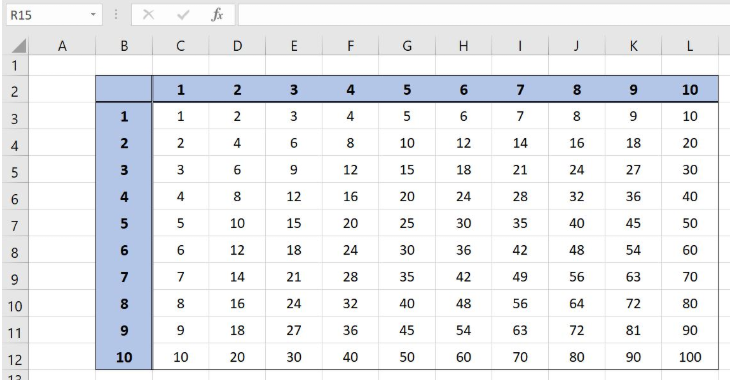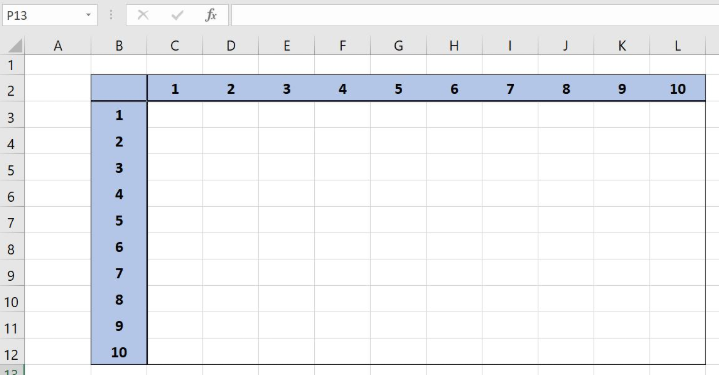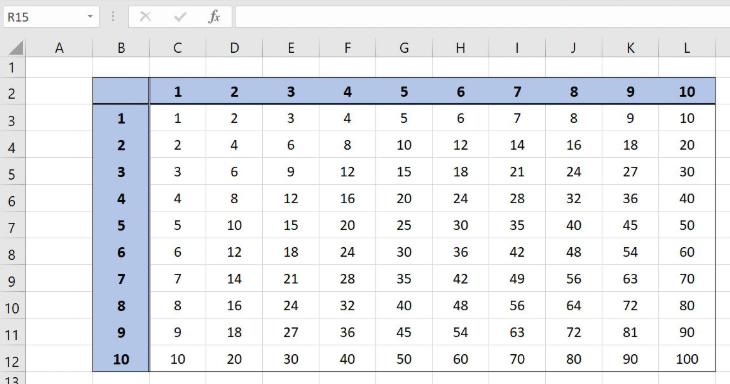Get instant live expert help with Excel or Google Sheets“My Excelchat expert helped me in less than 20 minutes, saving me what would have been 5 hours of work!”

#### Post your problem and you’ll get Expert help in seconds.

Your message must be at least 40 characters
Our professional Expert are available now. Your privacy is guaranteed.

# Multiplication table formula

Excel allows us to create a Multiplication table formula, using the simple multiplication and correct cell fixing. This step by step tutorial will assist all levels of Excel users in creating a multiplication table.Figure 1. Multiplication table formula

## Syntax of the Multiplication Table Formula

`=\$A1 * B\$1`

As we will copy the formula through the matrix of the cell, the fixing of the cells is very important. In the first parameter, we must fix the column and in the second the row. By setting the formula like this, we can easily copy the formula to the whole range and cells references will be changed accordingly.

## Setting up Our Data for Creating a Multiplication Table

Our table consists of numbers 1-10 in both first row and column. The results of the multiplication will be in the range C3:L12.Figure 2. Table structure for the multiplication table

## Creating the Multiplication table

First, we will create the formula in the cell C3 and then copy it to the rest of the cells.

The formula looks like:

`=\$B3 * C\$2`

To apply the formula, we need to follow these steps:

• Select cell C3 and click on it
• Insert the formula: `=``\$B3 * C\$2`
• Press enter
• Drag the formula down to the other cells in the column by clicking and dragging the little “+” icon at the bottom-right of the cell.
• Select the range C3:C12
• Drag the formula down to the other cells in the rows by clicking and dragging the little “+” icon at the bottom-right of the cell.Figure 3. Creating the Multiplication table

As you can see the result in C3 is 1, as multiplication of the values from B3 and C2. When we copy the formula to the other rows and columns, we get the full multiplication table.

Most of the time, the problem you will need to solve will be more complex than a simple application of a formula or function. If you want to save hours of research and frustration, try our live Excelchat service! Our Excel Experts are available 24/7 to answer any Excel question you may have. We guarantee a connection within 30 seconds and a customized solution within 20 minutes.

### Did this post not answer your question? Get a solution from connecting with the expert.Another blog reader asked this question today on Excelchat:## Subscribe to Excelchat.coAnother blog reader asked this question today on Excelchat: